# Force calculations

## Wire length L

If the geometry of the sample and the wire length are known the resultant angles and forces on the bonds during wire pull can be determined. If the wire length L is unknown, it can be calculated from the loop height.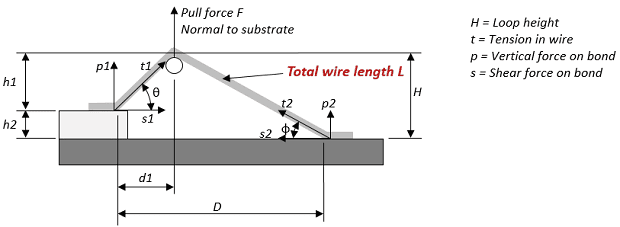The general equation is as follows: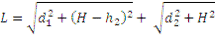If there is no height difference between the first and second bond and the pulltest is performed mid span, the equation can be simplified to: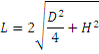## Position d1 from first bond angle θ

If you want to pull with a specific angle θ at the first bond, calculate the test position along the wire according to the following formula.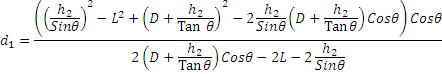## Position d1 from second bond angle θ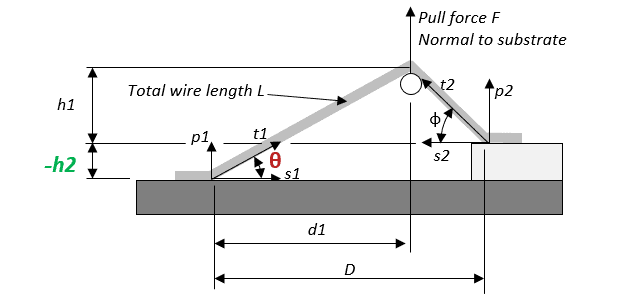You can use the same equation to calculate d1 for the second bond by making h2 negative. Φ then becomes θ and d1 is measured from the second bond.## Angle at second bond Φ

The angle at the second bond Φ is derived from this expression.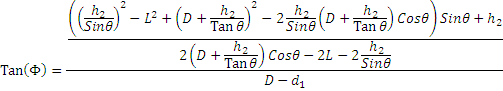## Pull at equal angles

If you want to pull with equal angles (θ=Φ), the following formulas apply.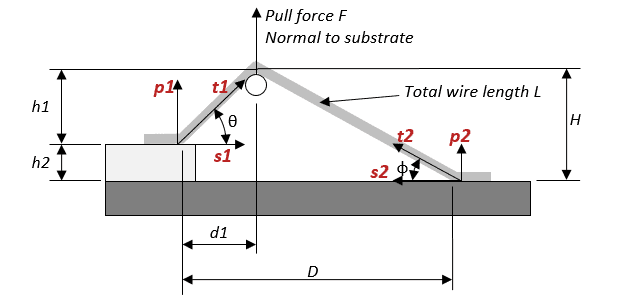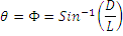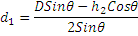The forces t, p and s then are as follows.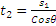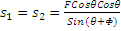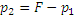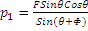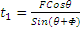Herbert Stuermann

Search